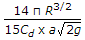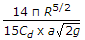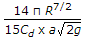# Mechanical Engineering - Hydraulics and Fluid Mechanics - Discussion

3.

A hemispherical tank of radius (R) has an orifice of cross-sectional area (a) at its bottom and is full of liquid. The time required to empty the tank completely is

 [A].[B].[C].[D].Answer: Option C

Explanation:

No answer description available for this question.

 Nehal said: (Feb 7, 2017) The units for the answer are wrong. The answer is option D.

 Muhammad Waqas said: (Feb 25, 2017) @Nehal Units are correct. Suppose unit of length is m (meter) and unit of time is s (second) then; Numerator units : m^(5/2). Denominator units: (m^2)(m^(1/2))(1/s^2)^1/2 = (m^5/2)(1/s). Numerator/demominator = m^(5/2) / m^(5/2)(1/s) = s.

 Bargav said: (Dec 11, 2018) Tell me the correct answer please.

 Akhileshwar said: (Mar 25, 2020) Unit wise option C is the Correct answer.

 Ganesh said: (May 1, 2021) Please tell me the correct answer.

#### Post your comments here:

Name *:

Email   : (optional)

» Your comments will be displayed only after manual approval.

#### Current Affairs 2021

Interview Questions and Answers